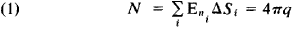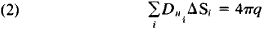Gauss Theorem

Gauss’ Theorem

a theorem of electrostatics proposed by K. Gauss and establishing the relation between a flux of intensity E of an electrical field through a closed surface having a charge q located within this surface. The product of the value of the surface element ΔSi, and the projection of En of vector E on the normal to ΔSi is called the flux of the vector E through the surface element ΔSi,. The flux N through closed surface S is equal to the sum of the fluxes through all surface elements. In absolute Gaussian units (cgs)Gauss’ theorem stems from Coulomb’s law—the law of the interaction of fixed point charges in a vacuum.

In a dielectric, Gauss’ theorem is valid for the flux of the vector of electrical induction D:where q is the total free charge within the surface S. Formula (2) represents the integral form of one of Maxwell’s equations for an electromagnetic field and expresses the fact that electrical charges are the sources of an electrical field.

G. IA. MIAKISHEV

Site: Follow: Share:
Open / Close